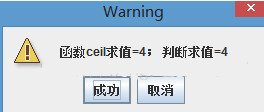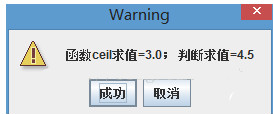# java取整函数，向上取整函数Math.ceil()

KLQ 2020-07-06 10:07:04 java常见问答 4785

java向上取整函数Math.ceil()：

```double dividend = 7; // 被除数
double divisor = 2; // 除数
double flag = 0;
int result1 = 0;
int result2 = 0;
// 函数式
flag = Math.ceil(dividend / divisor); //向上取整计算
result1 = (int) flag; //将结果转化为int数据类型
// 判断式：整除法
if ((dividend % divisor) == 0)
{
result2 = (int) dividend / (int) divisor; // 将操作数转化为int型数据
}
else
{
result2 = ((int) dividend / (int) divisor) + 1; // 将操作数转化为int型数据
}
Object[] options = {
"成功"
, "取消"
};
JOptionPane.showOptionDialog(null, "函数ceil求值=" + result1 + "； 判断求值=" + result2
, "Warning", JOptionPane.DEFAULT_OPTION
, JOptionPane.WARNING_MESSAGE, null, options, options);``````double dividend = 7; // 被除数
double divisor = 2; // 除数
double flag = 0;
int result1 = 0;
int result2 = 0;
// 函数式
// ① (int)dividend / (int)divisor 将计算结果直接转换为int型，因此这里的结果为3
//（int 类型丢失精度，即小数点之后的数丢失，本例的 3.5 丢失了0.5；
// 这里涉及 高精度数 向 低精度数 转化时，会丢失精度。
// 精度从低到高 int < double < float）.
// ② Math.ceil(3)函数执行，向上取整，也是3
// 感谢 博友“ws458371436” 的纠正，之前这个地方是糊涂的，还好有博友的细心，避免再误导其他朋友
flag = Math.ceil((int) dividend / (int) divisor); // 向上取整计算int = Math.ceil(int)，对int整数取整，纯属多余！
result1 = (int) flag; //将结果转化为int数据类型
// 判断式：整除法
if ((dividend % divisor) == 0)
{
result2 = dividend / divisor; // 保持double型数据类型
}
else
{
result2 = (dividend / divisor) + 1; // 保持double型数据类型
}
Object[] options = {
"成功"
, "取消"
};
JOptionPane.showOptionDialog(null, "函数ceil求值=" + result1 + "； 判断求值=" + result2
, "Warning", JOptionPane.DEFAULT_OPTION
, JOptionPane.WARNING_MESSAGE, null, options, options);```java取整函数的相关内容就给你介绍到这里啦!此文源于网络，仅供参考，希望可以对你有所帮助，更多java实例，可以继续关注本站来进行了解呢!

ajax回调函数怎么使用?ajax回调函数的使用方法

java怎么获取昨天,今天,明天的日期?java中获取日期的函数